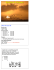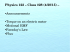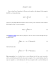# Homework #8: Magnetic Force and Biot-Savart Law

## Transcription

Homework #8: Magnetic Force and Biot-Savart Law
```Homework #8: Magnetic Force and Biot-Savart Law
1. [10 points] Suppose that an electron is released from rest in a region where there is a
uniform electric field in the z-direction and a magnetic field in the x-direction. Determine
the trajectory of the electron if it starts at the origin with velocity.
)̂
a. ⃗( ) (
)̂
b. ⃗( ) (
)( ̂
c. ⃗( ) (
̂)
2. Consider a rectangular loop of wire, supporting a mass m, which hangs vertically with
one end in a uniform magnetic field ⃗⃗, which points into the page.
a. [3 points] What current I in the loop would exactly balance the weight of the
mass?
b. [7 points] Suppose we now increase the current such that the magnetic force
exceeds the weight of the mass. This causes the loop to rise and thus, lifts the
mass. What is the work done to lift the wire? What force is doing work here?
3. Consider a circular wire loop of radius R located in the yz plane and carrying a steady
current I, as shown below.
a. [7 points] Calculate the magnetic field at an axial point P a distance x from the
center of the loop.
b. [3 points] Show that the magnitude of the magnetic field far from the loop is
given by
Here,
(
) is defined as the magnetic moment of the loop.
4. Magnetic Force Exerted on Current Carrying Loops
a. [5 points] Find the force on a square loop near an infinite straight wire. Assume
that both the loop and the wire carry a steady current I.
b. [5 points] Find the force on the triangular loop. Assume that the loop and wire
5. [10 points] A semicircular wire carries a steady current I as shown below. Show that the
magnetic field at a point P on the other semicircle is
[
(
)
( )
]
Bonus [7 points]: Using the Biot-Savart law, show that the magnetic field at a point
axis of the rotating sphere, as shown below, is given by
⃗⃗
Here,
̂
is the angular speed of a charge element around the z-axis
Hint: The following integrals may be helpful
∫ √
[(
∫
∫
√
)
) ]
(
√
(
)
on the
```

### PHYSQ 126, Quiz 6 (19 mars 2015) Interaction of a Current Loop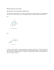### Ph 632 Problem Set #4 - Department of Physics | Oregon State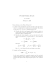### Problem Set 08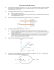### Physics 112 Sample Test 2 NAME __________________________### How to install RCS/RK TAGHeuer By Chronelec active loop### Problem Set 08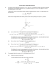### TEMPLATE HOW-TO MAKE A GUM PASTE BOW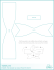### Worksheet/Problems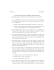### Formula sheet C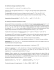### Physics 227 – Hourly Exam 2 ARC-103 (Aaa-Gzz), PLH (Haa-Lzz),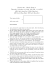### Medizinische Austellung A4 Wien 2008.indd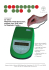### Homework #8: Magnetic Force and Biot-Savart Law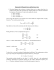### NATIONAL UNIVERSITY OF SINGAPORE DEPARTMENT OF PHYSICS ADVANCED PLACEMENT TEST (SAMPLE)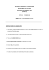### CBSE Sample Paper 6 1 General Instruction: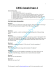### Review problems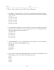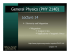### D - UCSB HEP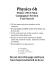### hemispheres t/t wire cover letter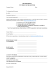### ME-709 Magnetic Recumbent Exercise Bike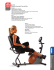### Review & Closure - Little Shop of Physics